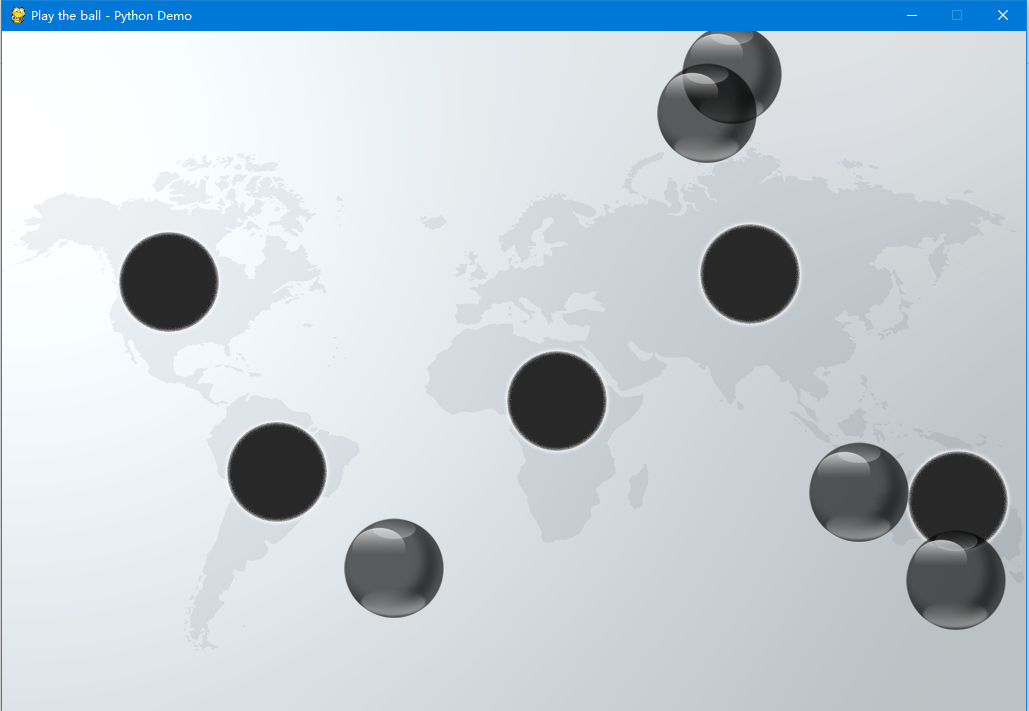# 《零基础入门学习Python》第085讲：Pygame：动画精灵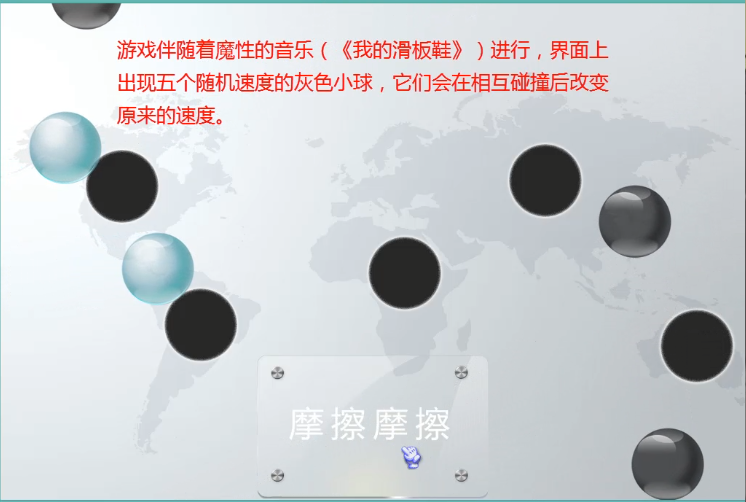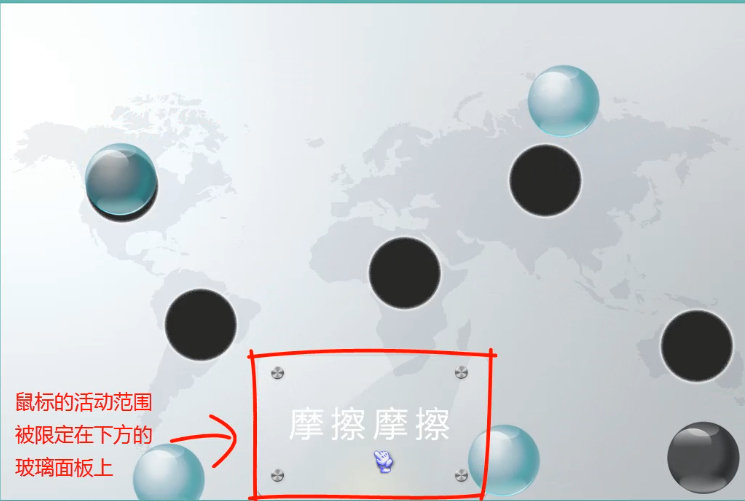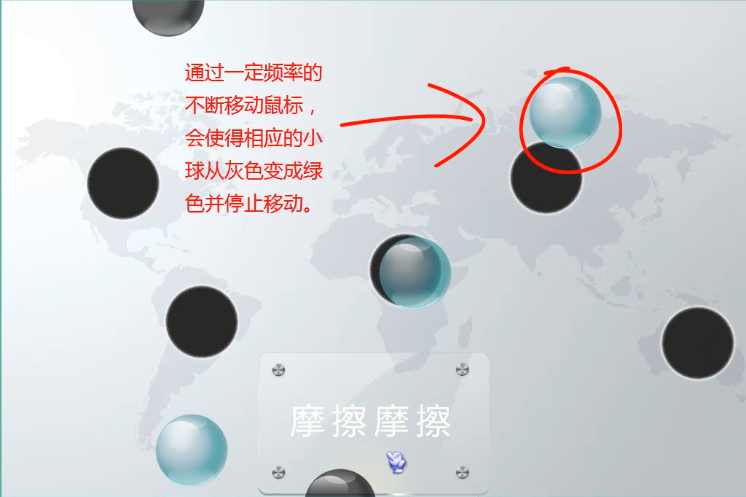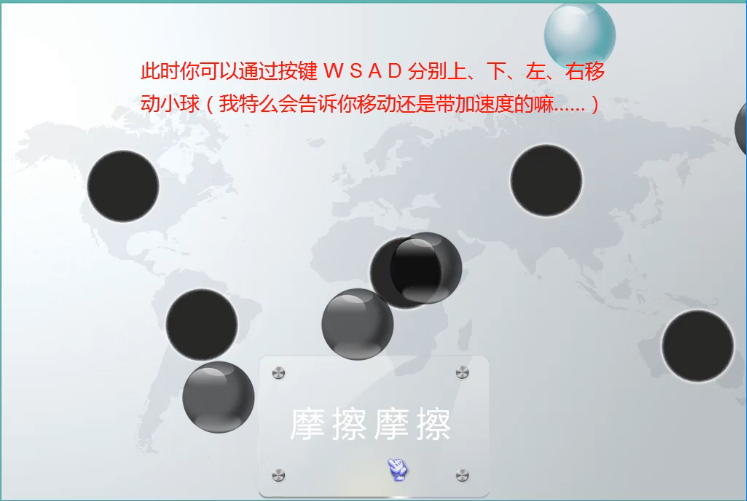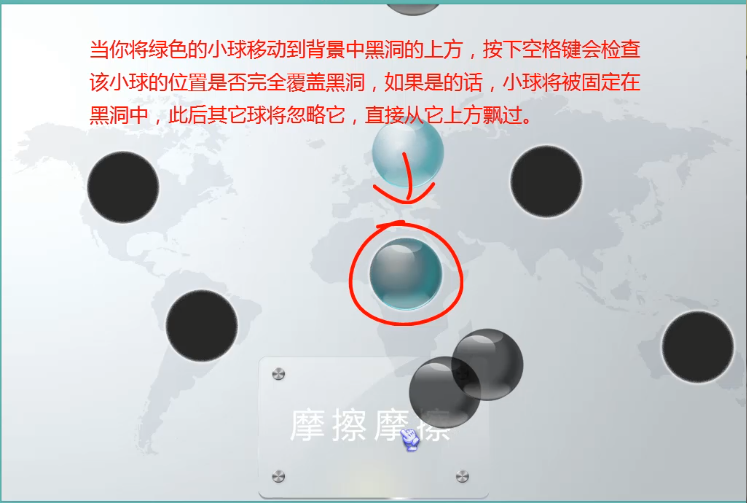#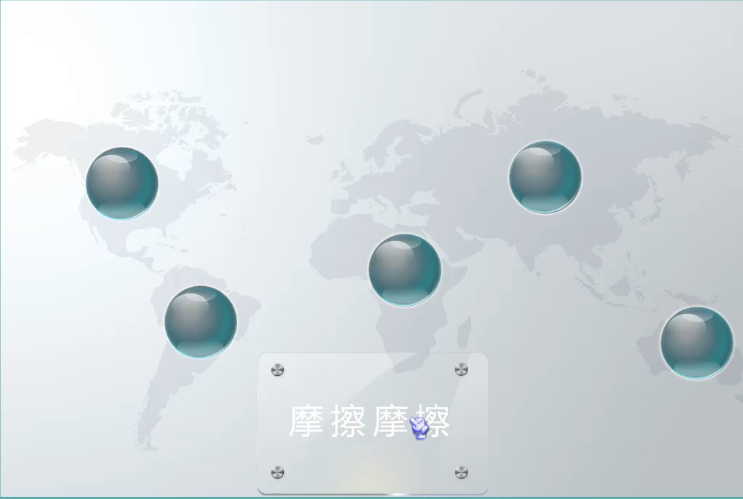至此，游戏结束！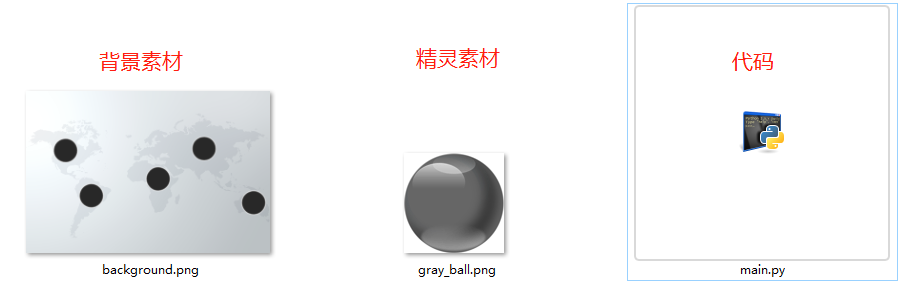import pygame
import sys
from pygame.locals import * #把Pygame 的常量名导进去
from random import *

class Ball(pygame.sprite.Sprite): #继承动画精灵基类
def __init__(self, image, position, speed, bg_size):
pygame.sprite.Sprite.__init__(self)

self.rect = self.image.get_rect()
self.rect.left, self.rect.top = position
self.speed = speed
self.width, self.height = bg_size, bg_size

def move(self):
self.rect = self.rect.move(self.speed)

#实现小球从某侧出界，从对侧进来
if self.rect.right < 0:
self.rect.left = self.width

elif self.rect.left > self.width:
self.rect.right = 0

elif self.rect.bottom < 0:
self.rect.top = self.height

elif self.rect.top > self.height:
self.rect.bottom = 0

def main():
pygame.init()

ball_image = "gray_ball.png"
bg_image = "background.png"

running = True

bg_size = width, height = 1024, 681
screen = pygame.display.set_mode(bg_size)
pygame.display.set_caption("Play the ball - Python Demo")

balls = []

for i in range(5): #创建5个球
position = randint(0, width-100), randint(0, height-100)
speed = [randint(-10, 10), randint(-10, 10)]
ball = Ball(ball_image, position, speed, bg_size)
balls.append(ball)

clock = pygame.time.Clock()

while running: #后面会在游戏结束时，给running 赋值 False。
for event in pygame.event.get():
if event.type == QUIT:  #当按关闭时停止游戏
sys.exit()

screen.blit(background, (0, 0))

for each in balls:
each.move()
screen.blit(each.image, each.rect)

pygame.display.flip()
clock.tick(30)

if __name__ == "__main__":
main()